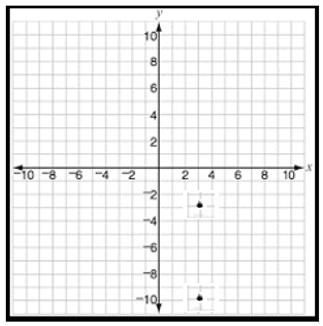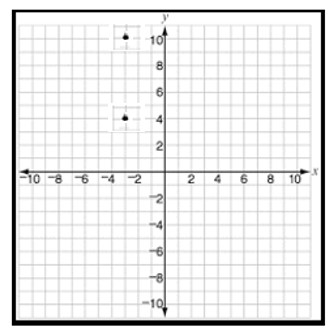# Finding distances between points that share a common coordinate given the graph

#### Complete Python Prime Pack

9 Courses     2 eBooks

#### Artificial Intelligence & Machine Learning Prime Pack

6 Courses     1 eBooks

#### Java Prime Pack

9 Courses     2 eBooks

In this lesson, we are given graphs with two points that share a common coordinate. We are required to find the distance between the two points in the graph.

### Rules to find distance between the points

• First we look at the coordinates of the two points in the given graph.

• We see that the two points have one of the coordinates same or have a shared common coordinate.

• The difference between the coordinates of the two points other than the shared coordinates gives the distance between the two points in the graph.

### Example

There be two points A and B on a grid. Find the distance between these two points A and B.

### Solution

Step 1 − Suppose the coordinates of points A and B are (5, 2), (9, 2). The shared common coordinates are the y coordinates.

Step 2 − So the distance these points is the difference between x coordinates 5 and 9, given by 9 – 5 = 4 units

Find the distance between the pair of points in the following graph −### Solution

Step 1 − The coordinates of the pair of points are (3, −3) and (3, −10).

Step 2 − The distance between the pair of points is the difference between their y coordinates as x coordinates are same.

Step 3 − The distance between the pair of points = −3 –(−10) = −3 + 10 = 7 units.

Find the distance between the pair of points in the following graph −### Solution

Step 1 − The coordinates of the pair of points are (−3, 10) and (−3, 4).

Step 2 − The distance between the pair of points is the difference between their y coordinates as x coordinates are same.

Step 3 − The distance between the pair of points = 10 – 4 = 6 units.